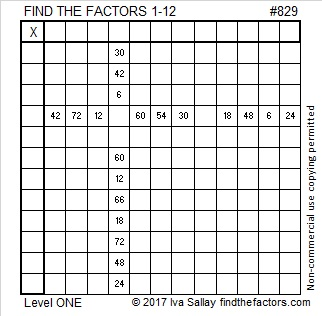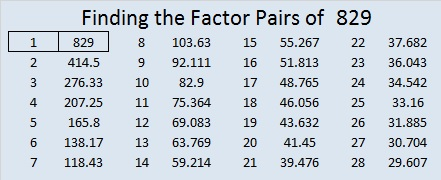# 829 Is the 24th Centered Triangular NumberPrint the puzzles or type the solution in this excel file: 12 factors 829-834

Whether you write today’s date as 8-15-17 or 15-8-17, today is (Primitive) Pythagorean Triple Day. 829 is also in a couple of primitive Pythagorean triples:

• 540 – 629 – 829, calculated from 2(27)(10), 27² – 10², 27² + 10²
• 829 – 343620 – 343621, calculated from (829)(1), (829² – 1²)/2, (829² + 1²)/2

829 is the last prime number in the fourth prime decade, (821, 823, 827, 829). Later this year we can celebrate the FIRST prime decade on 11-13-17 at 1900 hours.

Sara Van Der Werf wrote a post that includes a poster of these and other mathematical holidays that can be celebrated in the 2017-18 school year. She will also keep us posted on ways to celebrate these holidays as each one approaches. The poster is a word document that can be edited if need be.

829 is also the sum of three consecutive prime numbers: 271 + 277 + 281 = 829

829 is the 24th centered triangular number. I challenged myself to make a graphic that would show the significance of this fun number fact.Now you can see why it is called a CENTERED triangular number. If you count these concentric triangles including the tiny, barely visible one in the very center, you will see that there are 24 of them. Can you also see that 829 is equal to the sum of the 22nd, 23rd, and 24th triangular numbers?

What else is true because 829 is the 24th centered triangular number?

•  (22×23 + 23×24 + 24×25)/2 = 829.
• 3(23×24)/2 + 1 = 829

Here is 829’s factoring information:

• 829 is a prime number.
• Prime factorization: 829 is prime.
• The exponent of prime number 829 is 1. Adding 1 to that exponent we get (1 + 1) = 2. Therefore 829 has exactly 2 factors.
• Factors of 829: 1, 829
• Factor pairs: 829 = 1 × 829
• 829 has no square factors that allow its square root to be simplified. √829 ≈ 28.79236How do we know that 829 is a prime number? If 829 were not a prime number, then it would be divisible by at least one prime number less than or equal to √829 ≈ 28.8. Since 829 cannot be divided evenly by 2, 3, 5, 7, 11, 13, 17, 19, or 23, we know that 829 is a prime number.

Here’s another way we know that 829 is a prime number: Since its last two digits divided by 4 leave a remainder of 1, and 27² + 10² = 829 with 27 and 10 having no common prime factors, 829 will be prime unless it is divisible by a prime number Pythagorean triple hypotenuse less than or equal to √829 ≈ 28.8. Since 829 is not divisible by 5, 13, or 17, we know that 829 is a prime number.This site uses Akismet to reduce spam. Learn how your comment data is processed.